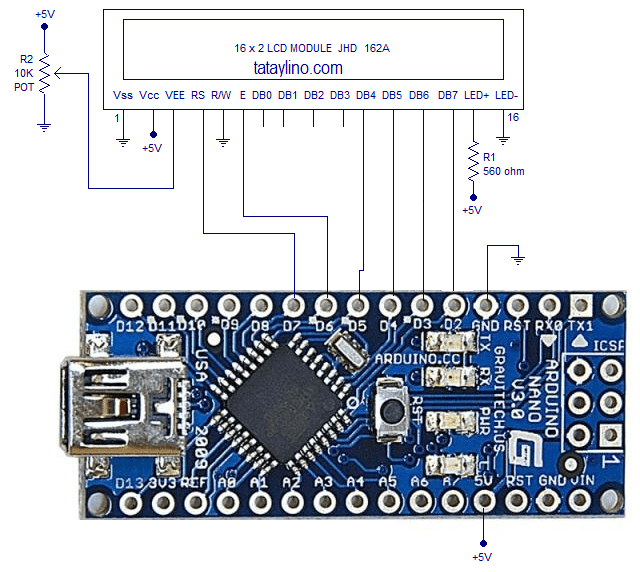# Connecting Arduino Nano to 16×2 LCDConnecting arduino to 16×2 LCD is very much easy compared to microchips PIC microcontrollers. This maybe because the arduino has lots of software library aimed for beginners. The program is easy to understand, I am sure you can be able to understand it even if you are still new to programming.

schematic

This use the 4-bit mode of the LCD to save some pins of the arduino so you can maximize the pin for other purposes. The 4-bit mode enables us to use just 6pin of the arduino instead of 11pins.Program

The program is simple and straightforward.

[code lang=”c”]
// include the library code:
#include <LiquidCrystal.h> // initialize the library with the numbers of the interface pins – The numbers
//are the pin connected in sequence from RS to DB7
LiquidCrystal lcd(7, 6, 5, 4, 3, 2);

void setup()
{
// set up the LCD’s number of columns and rows:
lcd.begin(16, 2);
// Print a message to the LCD
lcd.print(“hello, world!”);

}void loop() {
// set the cursor to column 0, line 1
// (note: line 1 is the second row, since counting begins with 0):
lcd.setCursor(0, 1);
// print the number of seconds since reset:
lcd.print(“tataylino.com”);
}
[/code]

```Update: You may encounter problem on compiling on line with "lcd.print" command.
The simple solution is just re-type this line. Don't copy and paste it.```